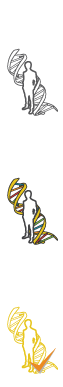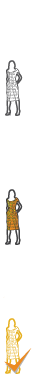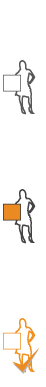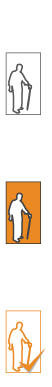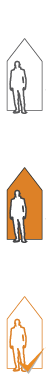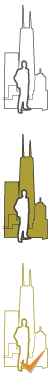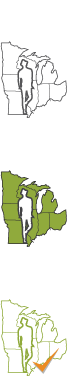#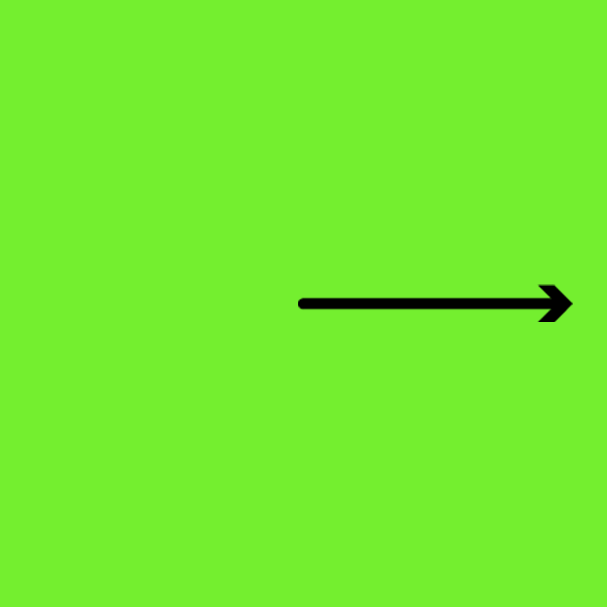Angles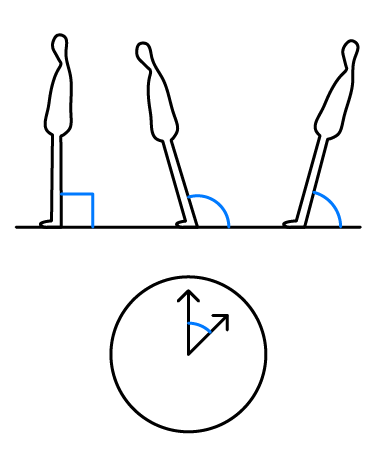What are angles? An angle starts with two straight lines that meet at a common point. The point is called a vertex. The Latin word ‘angulus,’ means “corner.” The measure of degrees between the two lines is considered the angle. For example, if you stand up in a room in your house, the intersection of the plane of the floor and the line of you standing on it create a 90-degree angle. If you stand with your legs and arms apart in the shape of an “x”, your legs create an angle and the space between your arms does, too. Look down at your hand. Open your fingers. Between each two fingers is an angle! Find some scissors. Open the scissors a little and you will see an angle between the two blades; open the scissors wider and you will a wider angle! Find a wall clock or a watch. You will see the hour hand and the minute hand as they tick away the time. They form an angle. Angles are all around us! Become an angle explorer.

## Activity 1 – Measuring Angles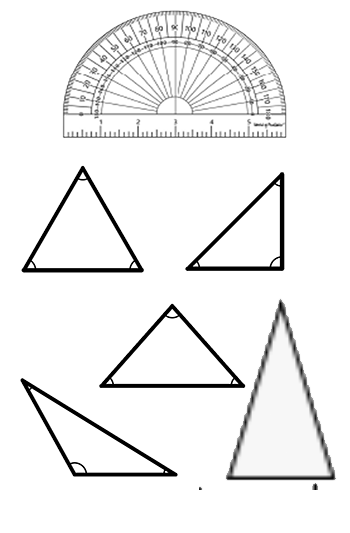As you discovered in the introduction, angles can be narrow and they can be wide. The flat floor is actually a 180-degree angle. If you lie down on the floor and lift your legs straight up, you will form a 90-degree angle. If you lay down, someone can draw a half circle starting by at the end point of your outstretched arms over your waist and to your feet and create a 180-degree circle. If you flap your arms and legs open and closed (like making a snow angel) you can mark a complete circle of 360 degrees.

Think again of the clock. The hands move 360 degrees around a circle. If you divide the circle into half you will have two 180-degree half circles; if you divide the half circles into half you will have four quarters or four 90-degree angles. Now divide the circle into thirds, fifths, and sixths. Using a protractor can help you divide a circle into halves, thirds, fourths, and so on. The equation to find the angle degrees for each of these will be the angle in degrees = 360 degrees of a circle divided by 3 (120 degrees), divided by 5 (72 degrees), and divided by 6 (60 degrees). Draw 4 circles divided in half, divided in 4ths, divided in 5ths, and divided in 6ths. Upload them to the gallery.

## Activity 2 – Types of Angles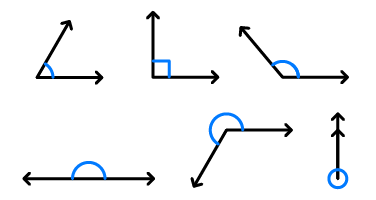If an angle is narrower than 90 degrees, it is called an acute angle. If it is between 90 and 180 degrees it is called an obtuse angle. If it is 180 degrees, it is called a straight angle. If it is between 180 and 360 degrees it is called a reflex angle. If it is 360 degrees it is called a complete angle. Draw and label all of the types of angles. A reflex angle is an angle that measures between 180˚ and 360. Draw and label an acute angle, an obtuse angle, a straight angle, a reflex angle, and a full angle. Know your angles!

## Activity 3 – Triangles and Inside and outside angles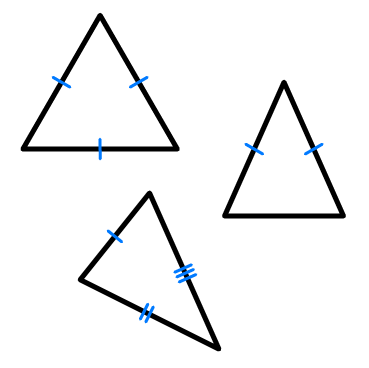A triangle is a three-sided polygon. All triangles have three sides and three angles. The triangle’s three interior angles all add up to 180 degrees. Think of the pyramids. Draw three different heights of pyramids. Draw one that has a narrow base and two tall sides. Now draw a triangle that has a very wide base and is shorter than the narrow base triangle. Draw another that has three equal sides and three equal angles. This triangle is an equilateral triangle meaning that all sides and angles are equal to each other. Using a protractor, measure the inside and outside angles at each vertex and label them. The one with equal inside angles is called an Equilateral Triangle. A Scalene triangle is a triangle where all sides and all angles are different lengths or different degrees. An Isosceles Triangle is a triangle that has two equal sides of three, and two equal angles of three. Draw three different triangles and label their type.

## Review

• The point where two straight lines meet together to become an angle is called a vertex.
• 360 degrees make up a circle.
• What kind of angle is narrower than 90°? - acute, obtuse, straight, reflex
• What kind of triangle is made up of three equal sides? - equilateral, scalene, isosceles
• All triangles interior angles add up to 180°.